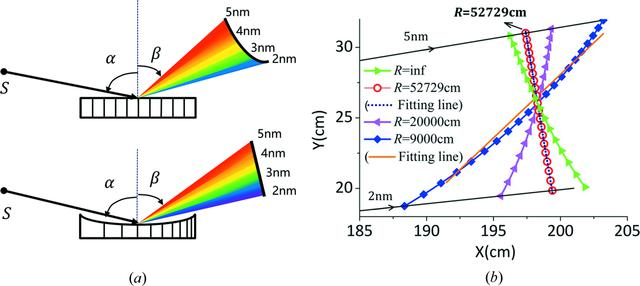disable zoom     view article Figure 1 (a) A schematic diagram of a VLS grating on a concave substrate to achieve an excellent flat-field condition in the meridional plane within the spectral range 2–5 nm (bottom) is compared with a plane grating with constant groove density whose focal spots for the same spectral range lie in a curved line (top). (b) A change of the meridional focal curves of the spectrometer is observed when applying different meridional radii R, while the identical parameters are as follows: D0 = 24000 lines cm−1, rm = 1000 cm,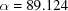° and the image focal length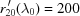cm at the center wavelength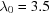nm. The straight fitting lines represent the best detector plane for each R, where the value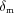is the magnitude of `defocus' over the whole spectral range [defined in §2.1 and equation (10)]. The fitting lines for R = 9000 and 52729 cm are depicted, while the diffraction beams of 2 nm and 5 nm are shown simultaneously. The calculated valuefor each case is: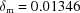cm (R = infinity),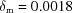cm (R = 52729 cm),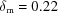cm (R = 20000 cm),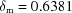cm (R = 9000 cm).JOURNAL OFSYNCHROTRONRADIATION
ISSN: 1600-5775
Volume 25| Part 3| May 2018| Pages 738-747